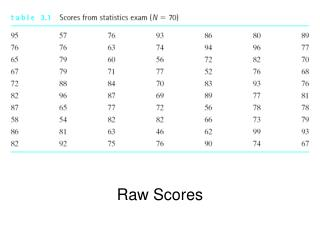DownloadDownload PresentationRaw Scores

# Raw Scores

Télécharger la présentation## Raw Scores

- - - - - - - - - - - - - - - - - - - - - - - - - - - E N D - - - - - - - - - - - - - - - - - - - - - - - - - - -
##### Presentation Transcript

1. Raw Scores

2. Un-Grouped Frequency Distribution

3. Grouped Frequency Distribution

4. Percentile and Percentile Rank • Percentile (PX) or Percentile Point • The value on the measurement scale (at or) below which a specified percentage of the scores in the distribution fall. • Where x from Px is a percentage • Looking for a score (value) • Percentile Rank (PRX) • The percentage of scores with values (equal or) lower than the score in question. • Where x from PRx is a score (value) • Looking for a percentage

5. Table 3.7 (p. 46) Relative frequency, cumulative frequency, and cumulative percentage distributions… Note. The Relative fs were removed from this table since they are not required to calculate percentiles and/or percentile ranks. Determine (compute) the 50th Percentile (P50) for the statistics exam scores.

6. Table 3.7 (p. 46) Relative frequency, cumulative frequency, and cumulative percentage distributions… Note. The Relative fs were removed from this table since they are not required to calculate percentiles and/or percentile ranks. Determine (compute) the 20th Percentile (P20) for the statistics exam scores.

7. Table 3.7 (p. 46) Relative frequency, cumulative frequency, and cumulative percentage distributions… Note. The Relative fs were removed from this table since they are not required to calculate percentiles and/or percentile ranks. Determine (compute) the 75th Percentile (P75) for the statistics exam scores.

8. Table 3.7 (p. 46) Relative frequency, cumulative frequency, and cumulative percentage distributions… Note. The Relative fs were removed from this table since they are not required to calculate percentiles and/or percentile ranks. Determine (compute) the percentile rank (PR) for a score of 86.

9. Table 3.7 (p. 46) Relative frequency, cumulative frequency, and cumulative percentage distributions… Note. The Relative fs were removed from this table since they are not required to calculate percentiles and/or percentile ranks. Determine (compute) the percentile rank (PR) for a score of 59.

10. Graphing Data Constructing a Graph

11. Distorting Data Through Graphing…

12. Distorting Data Through Graphing…

13. Frequency distributions of nominal or ordinal (categorical) data are customarily plotted using the bar graph.

14. Frequency distributions of interval or ratio (continuous) data are customarily plotted using the histogram.

15. SHAPES of Frequency Curves

16. Given the following set of scores, determine the stem and leaf for the 30s: 10 31 25 35 30 39 45 36 36 68 39 32